Drive_Index

Critical (Whirling) Speed Of Shafts

Introduction

For a rotating shaft there is a speed at which, for any small initial deflection, the centripetral force is equal to the elastic restoring force.  At this point the deflection increases greatly and the shaft is said to "whirl".  Below and above this speed this effect is very much reduced.  This critical (whirling speed) is dependent on the shaft dimensions, the shaft material and the shaft loads .  The critical speed is the same as the frequency of traverse vibrations.

The critical speed Ncof a shaft is simply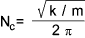Where m = the mass of the shaft assumed concentrated at single point .
k is the stiffness of the shaft to traverse vibrations

For a horizontal shaft this can be expressed as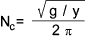Where y = the static deflection at the location of the concentrated mass

Symbols
 m = Mass (kg) Nc = critical speed (rev/s ) g = acceleration due to gravity (m.s-2 ) O = centroid location G = Centre of Gravity location L = Length of shaft E = Young's Modulus (N/m2) I = Second Moment of Area (m4) y = deflection from δ with shaft rotation = ω δ static deflection (m) ω = angular velocity of shaft (rads/s)

Theory

Consider a rotating horizontal shaft with a central mass (m) which has a centre of gravity (G)slightly away from the geometric centroid(O)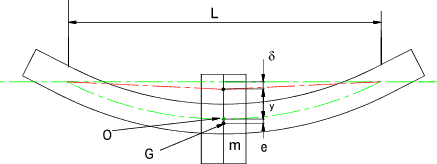The centrifugal force on the shaft = m ω 2(y + e) and the inward pull exerted by the shaft = y (48EI / L 3).
Reference Beam theory - Deflection
The more general formulea for the restoring traverse force of the beam is y (K EI / L 3) where K = a constant depending on the position of the mass and the end fixing conditions.

Equating these forces...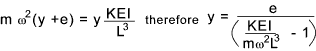When the denominator = 0 ,that is [ KEI / m ω 2 L 3 ] = 1 , the deflection becomes infinite and whirling takes place.

The whirling or critical speed is therefore.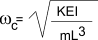For a simply supported beam with a central mass K = 48 .. See examples below

Substituting ω c 2 for KEI /mL3 in the above equation for y results in the following equation which relates the angular velocity with the deflection.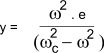This is plotted below..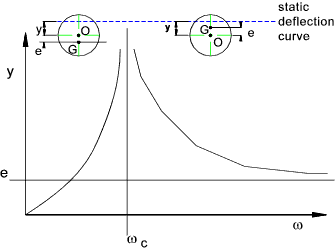This curve shows the deflection of the shaft (from the static deflection position) at any speed ω in terms of the critical speed.

When ω < ωc the deflection y and e have the same sign that is G lies outside of O.  When ω > ωc then y and e are of opposite signs and G lies between the centre of the rotating shaft and the static deflection curve.  At high speed G will move such that it tends to coincide with the static deflection curve.

Cantilever rotating mass

Mass of shaft neglected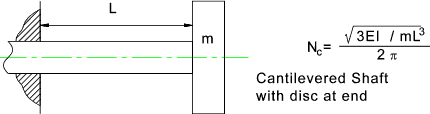Central rotating mass- Long Bearings

Mass of shaft neglected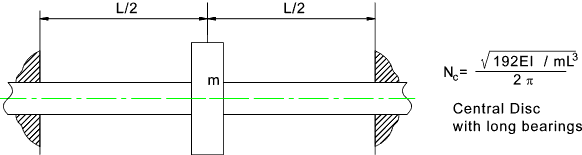Central rotating mass - Short Bearings

Mass of shaft neglected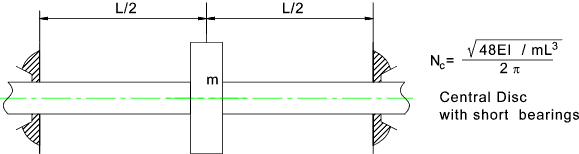Non-Central rotating mass - Short Bearings

Mass of shaft neglected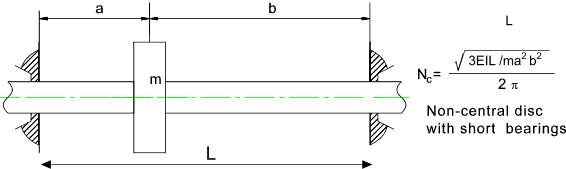Cantilevered Shaft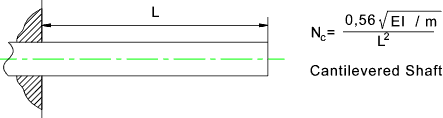m = mass /unit length

Shaft Between short bearings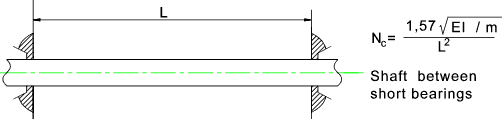m = mass /unit length

Shaft Between long bearings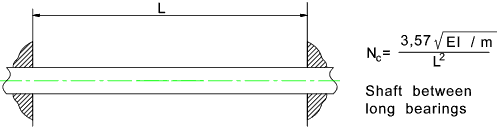m = mass /unit length

This is known as Dunkerley's method an is based on the theory of superposition....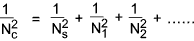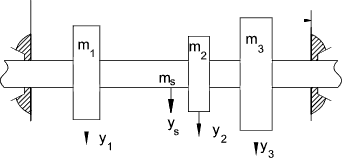Links to Information on designing shaft suppliers and shaft locking methods Mitcalc-Shafts ..Notes associated with spreadsheet calculator - very useful on their own Critical speeds - Distributed Loads ..Useful Webpage from~EngnieersEdge Critical speeds - Wikipedia ..Detailed notes

Drive_Index